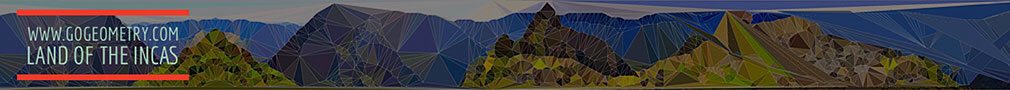# Nine-Point Center, Nine-Point Circle, Euler Line, Step by Step Interactive Illustration. Orthocenter, Circumcenter, Centroid, Midpoint. HTML5 Animation. Click the Next button below to start.The Nine-point center is the center of the nine-point circle. The Nine-Point Circle of triangle ABC with orthocenter H is the circle that  passes through the feet of the altitudes HA, HB and HC to the three sides, the midpoints MA, MB and MC of those sides, and the Euler Points EA, EB and EC, which are the midpoints of the segments AH, BH, and CH, respectively. Euler line is the line passing through the orthocenter H, the nine-point center N, the centroid G, and the circumcenter O of any triangle ABC. Click the Next button above to start the step-by-step illustration. The nine-point center N is the midpoint of the line HO. The distance from the orthocenter H to the centroid G is twice the distance from the circumcenter O to the centroid G. The nine-point center N is the circumcenter of the medial triangle MAMBMC. The nine-point center N is the circumcenter of the orthic triangle HAHBHC. The nine-point circle is also known as Euler's circle and Feuerbach's circle. Leonhard Euler showed in 1765 that the nine-point circle bisects any line from the orthocenter to a point on the circumcircle. In 1822 Karl Feuerbach discovered that any triangle's nine-point circle is externally tangent to that triangle's three excircles and internally tangent to its incircle. See Also:Nine-Point Center, Nine-Point Circle, Euler Line, HTML5 Animation for iPad. Circumcenter, Centroid, Orthocenter. GeoGebra, Dynamic Geometry: Internal Feuerbach Point HTML5 Animation for Tablets (iPad, Nexus) Geometry Problem 1361. Triangle, Two Nine-Point Circles, Feuerbach's Circle, Euler's Circle, Congruent Angles, Sketch, iPad Apps. Geometry Problem 1360. Triangle, Nine-Point Circle, Feuerbach's Circle, Euler's Circle, Cyclic Quadrilateral, Concyclic Points, Sketch, iPad Apps. Geometry Problem 1062. Scalene Triangle with an angle of 60 degrees, Euler Line, Orthocenter, Circumcenter, Distance, Congruence, Transversal. Geometry Problem 761 Scalene Triangle with an angle of 60 degrees, Euler Line, Equilateral Triangle. Circumcenter. Index. Centroid /Barycenter. Index. Orthocenter Index. iPad Apps: Apollonius Software. Feuerbach Point. Triangle, Incircle, Nine point circle, Tangent circles, Orthocenter, Midpoint. Interactive Geometry Software.

 Home | Sitemap | Search | Geometry | HTML5 and Geometry | Triangle Centers | Orthic Triangle | Visual Index | HTML5 Animation for iPad and Nexus | Email | Post a comment | by Antonio Gutierrez Last updated: Jul 12, 2018